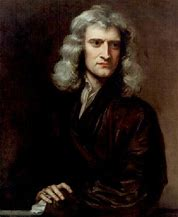```WP_Term Object
(
[term_id] => 6435
[name] => AI
[slug] => artificial-intelligence
[term_group] => 0
[term_taxonomy_id] => 6435
[taxonomy] => category
[description] => Artificial Intelligence
[parent] => 0
[count] => 404
[filter] => raw
[cat_ID] => 6435
[category_count] => 404
[category_description] => Artificial Intelligence
[cat_name] => AI
[category_nicename] => artificial-intelligence
[category_parent] => 0
)
```

# Machine Learning with Prior Knowledge

Machine Learning with Prior Knowledge
by Bernard Murphy on 08-09-2018 at 7:00 am

I commented recently on limitations in deep learning (DL), one of which is the inability to incorporate prior knowledge, like basic laws of mathematics or physics. Typically, understanding in DL must be inferred from the training set, which in a general sense cannot practically cover prior knowledge. Indeed one of the selling points of DL is that it doesn’t need to be programmed with algorithms; intelligence is inferred entirely from these training sets through a form of optimization. This works well when the training set is large enough to cover the most important aspects of an objective but not so well when other variations are introduced, such as rotations or movement. That’s a rather big limitation.The brute-force way to solve this problem is to expand the training to cover more variations. For rotations, instead of N training samples, perhaps you need 108*N to cover 3 axes of rotation and 36 orientations in each axis (0, 10, 20, … degrees). That’s a massive increase in the number of training samples you have to gather and label. For movement, how do you train ML to determine what will happen to all the other balls on a snooker table when you strike the cue ball? Using training to rediscover what Newton codified over 300 years ago seems like a huge waste of ingenuity.

The best way to handle these variants is to use prior knowledge in math and physics, combined with ML. In computer graphics we infer the impact of rotations on a view using algorithms based on math formulae. In the snooker example, we use Newton’s laws of motion, again encoded in algorithms. Those laws/algorithms capture in a few simple equations what would otherwise require perversely large training sets in the pursuit of algorithm-free recognition. So much for banishing algorithms.

One paper from Stanford uses an understanding of projectile mechanics to identify and track the path of a pillow thrown across a room. As far as I can tell, they model recognition in short segments of the path first, then use constraints to penalize complete paths which don’t follow the expected second-order equation of motion. In effect they are using a classical formula as a constraint in the structure of a neural net. This work shows some promise for learning in such contexts with only weak supervision.

Another interesting paper from the Institute of Science and Technology in Austria takes a different approach to build (through ML) models for safe operating conditions for robots (such as ranges of moving arms or legs) based on learning simple formulae from operations in a known-safe range. These formulae then allow extrapolations beyond the trained range. They describe this as “a machine learning method to accurately extrapolate to unseen situations”, in effect building its own prior knowledge in the form of simple linear equations through experiments in a more bounded space.

A third example from the Sorbonne University provides an illustration of forecasting sea-surface temperatures (SSTs). Surface temperature data is already generated through satellite imagery, providing vast amounts of current and historical information. Forecasting how this will develop requires evolving this data forward in time based on partial differential equations (PDEs) and is the basis for the standard approach to forecasting using numerical solution methods. This research team instead uses a CDNN with discretized version of the PDE equations to guide weighting in time-propagation in the net. Their work shows promising results in comparison with numerical methods and some other NN approaches.

So, two methods which reduce/discretize prior knowledge (physics) to mechanisms which fit into existing deep learning architectures through weighting and one which derives simple equations to form its own “prior” base of knowledge. Intriguing directions, though for me the Sorbonne approach seems the most extensible since almost all problems in physics can be reduced to PDEs (though I guess the geometry of present-day neural nets will limit application to 2 dimensions, plus time).